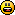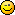## News:

*NEW* Current Version on STEAM16.793

Webchat:
Visit the chat

## 3D GRAVITY!

#### Hemlos

June 14, 2012:
I attached a 3d galactic simulator to this message...play with the file called "Profile"...youll be able to affect the gravity and shape of the formations.
I made a bunch of different formations..these buttons will start them:
`1234567890-=[backspace]

I APPOLOGIZE from the bottom of my heart, really, im sorry for not keeping up with this thread.
It is sloppy, on the fly recoding, to keep up with the IDE...i have too much on my plate.
If you need help with your own program handling 3d gravity, i can help you by reviewing code.

Here is how you do 3d gravity...
Basically...i just used isaac newtons formula on each dimension, then divided by the number of dimensions.
You must alter the ALL forces on every object, which is induced by all other objects with mass.
In other words...if theres 100 objects...you must change the force applied to each object, 99 times(indices of all masses affect each one)
...and then do it for the second(third, fourth,etc) object...so this would be 100x99 loops(not 100x100 because you dont calculate the gravity of an object on itself X)

In order to explain the gravity, i ripped the most important code and splattered it here below as neatly as i could:
Code (glbasic) Select
`//Predefine Gravity and masses:G=1.615 //Gravitational constant...this must not change. The value can be anything you want.//All objects must have predefined MASS(size), and Position.// In other words, one object might have the mass of 3 while another object can be size of 10.//so you begin with a loop calculating each object separately://You apply all the forces of all objects onto the first object.....then again for the second and third...etcM = mass of the object 1//xyz of object, which gravity force will be applied to:X=ObjPos[Object1] // xyz of Object(next in indices) being affectedY=ObjPos[Object1]Z=ObjPos[Object1]//this is the beginning of the SECOND loop...apply force to first object according to all the other forces in the indices//Define position of next objects force://Object #2 xyz position (next XYZ)nX=ObjPos[Object2] // xyz of Obj2 of next object in listnY=ObjPos[Object2]nZ=ObjPos[Object2]//vector distance(Range)://R must be > 1...i limited my test to 1.001 as a minimum..or else i forced a collision event here.//You will experience a [numerical terminal velocity] of objects colliding, when the vector distance drops to 1.0RX=(MAX(X,nX)-MIN(X,nX))RY=(MAX(Y,nY)-MIN(Y,nY))RZ=(MAX(Z,nZ)-MIN(Z,nZ))R2=(RX*RX)+(RY*RY)+(RZ*RZ)R=SQR(R2)R3=(R*R*R)//Now you must apply the gravity to the force being put on the first object(XYZ affected by xforce)// you keep altering this xforce until all indices are read.Gravity=G*M2 //this is force being applied to the current force...not really gravity..variables need names.IF X>nX THEN XForce=XForce-Gravity*RX/R3IF X<nX THEN XForce=XForce+Gravity*RX/R3IF Y>nY THEN YForce=YForce-Gravity*RY/R3IF Y<nY THEN YForce=YForce+Gravity*RY/R3IF Z>nZ THEN ZForce=ZForce-Gravity*RZ/R3IF Z<nZ THEN ZForce=ZForce+Gravity*RZ/R3//end of loop....by this point, first object will calculated, because you applied the forcee from all other objects on the first one//the loop will now run through and affect the force applied to the second object in the indices of objects.// after all objects are calculated//now you must move the object according to the forces applied onto it:ObjPos[Object1]=X+XForceObjPos[Object1]=Y+YForceObjPos[Object1]=Z+ZForce//and dont forget, you must record into memory the force applied onto the object you are moving here.//this data will be used again....in the next program frame.ObjPos[Object1]=XForceObjPos[Object1]=YForceObjPos[Object1]=ZForce`

I know..its hard to understand...im not a very good teacher, but someone might 'get it'.

Bing ChatGpt is pretty smart#### Kitty Hello

#1
Waiting impatiently ....#### Quentin

#2
there will be at least one lucky guy looking for 2D gravity as 3D is still a mystery for me#### Hb!

#3
Wow please post the 2d gravity code as well . i want to apply them to my sprites... tsk you saved me a lot of work i was going o search that formula... but I saw your post just in time XD....

#### Hemlos

#4
Bing ChatGpt is pretty smart